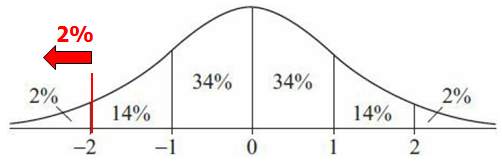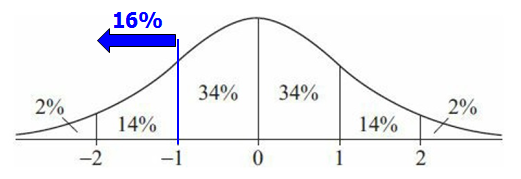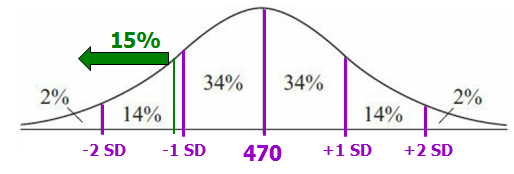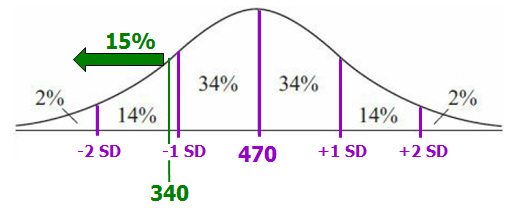It is currently 20 Jun 2019, 11:08GMAT Club Daily Prep

Thank you for using the timer - this advanced tool can estimate your performance and suggest more practice questions. We have subscribed you to Daily Prep Questions via email.

Customized
for You

we will pick new questions that match your level based on your Timer History

Track

every week, we’ll send you an estimated GMAT score based on your performance

Practice
Pays

we will pick new questions that match your level based on your Timer History

Not interested in getting valuable practice questions and articles delivered to your email? No problem, unsubscribe here.QOTD#13 The figure above shows the standard normalQuestion banks Downloads My Bookmarks Reviews Important topics
Author Message
TAGS:GRE Prep Club LegendJoined: 07 Jun 2014
Posts: 4810
GRE 1: Q167 V156WE: Business Development (Energy and Utilities)
Followers: 118

Kudos [?]: 1899  , given: 397

QOTD#13 The figure above shows the standard normal [#permalink]
4
KUDOS
Expert's post00:00

Question Stats:62% (01:00) correct37% (01:24) wrongbased on 35 sessions
Attachment:#GREpracticequestion The figure above shows the standard normal distribution.jpg [ 27.95 KiB | Viewed 2662 times ]

The figure above shows the standard normal distribution, with mean 0 and standard deviation 1, including approximate percents of the distribution corresponding to the six regions shown.

The random variable Y is normally distributed with a mean of 470, and the value Y = 340 is at the 15th percentile of the distribution. Of the following, which is the best estimate of the standard deviation of the distribution?

A. 125
B. 135
C. 145
D. 155
E. 165

Practice Questions
Question: 15
Page: 152
[Reveal] Spoiler: OA

_________________

Sandy
If you found this post useful, please let me know by pressing the Kudos Button

Try our free Online GRE TestGRE Prep Club LegendJoined: 07 Jun 2014
Posts: 4810
GRE 1: Q167 V156WE: Business Development (Energy and Utilities)
Followers: 118

Kudos [?]: 1899  , given: 397

Re: QOTD#13 The figure above shows the standard normal [#permalink]
1
KUDOS
Expert's post
Explanation

Since you know that the distribution of the random variable Y is normal with a mean of 470 and that the value 340 is at the 15th percentile of the distribution, you can estimate the standard deviation of the distribution of Y using the standard normal distribution. You can do this because the percent distributions of all normal distributions are the same in the following respect: The percentiles of every normal distribution are related to its standard deviation in exactly the same way as the percentiles of the standard normal distribution are related to its standard deviation.

For example, approximately 14% of every normal distribution is between 1 and 2 standard deviations above the mean, just as the figure above illustrates for the standard normal distribution.

From the figure, approximately 2% + 14%, or 16%, of the standard normal distribution is less than –1. Since 15% < 16%, the 15th percentile of the distribution is at a value slightly below –1. For the standard normal distribution, the value –1 represents 1 standard deviation below the mean of 0. You can conclude that the 15th percentile of every normal distribution is at a value slightly below 1 standard deviation below the mean.

For the normal distribution of Y, the 15th percentile is 340, which is slightly below 1 standard deviation below the mean of 470. Consequently, the difference 470 – 340, or 130, is a little greater than 1 standard deviation of Y; that is, the standard deviation of Y is a little less than 130. Of the answer choices given, the best estimate is 125, since it is close to, but a little less than, 130. The correct answer is Choice A.
_________________

Sandy
If you found this post useful, please let me know by pressing the Kudos Button

Try our free Online GRE TestGRE InstructorJoined: 10 Apr 2015
Posts: 1995
Followers: 60

Kudos [?]: 1811  , given: 9

Re: QOTD#13 The figure above shows the standard normal [#permalink]
7
KUDOS
Expert's post
sandy wrote:The figure above shows the standard normal distribution, with mean 0 and standard deviation 1, including approximate percents of the distribution corresponding to the six regions shown.

The random variable Y is normally distributed with a mean of 470, and the value Y = 340 is at the 15th percentile of the distribution. Of the following, which is the best estimate of the standard deviation of the distribution?

A. 125
B. 135
C. 145
D. 155
E. 165

Tricky!!!

First recognize that about 2% of the entire population is MORE THAN 2 units of standard deviation less than the mean.
In other words, if a value is 2 units of standard deviation below the mean, then that value is at the 2nd percentile.Also recognize that about 16% of the entire population is MORE THAN 1 unit of standard deviation less than the mean.
In other words, if a value is 1 unit of standard deviation below the mean, then that value is at the 16th percentile.So, a value that's at the 15th percentile will be a little more than 1 unit of standard deviation less than the mean.We're told that a certain normally distribution has a mean of 470.
So, it will look like this:We're also told that 340 is at the 15th percentile of the distribution. So, we'll add that here:Notice that the value associated with the 15th percentile is MORE THAN 1 standard deviation below the mean.

If we had to approximate, we might say that 340 is 1.1 unit of standard deviation below the mean.
NOTE: This approximation is not that important, as long as we recognize that 340 is MORE THAN 1 standard deviation below the mean. You'll see why this is shortly.

The mean = 470, which means 340 is 130 less than the mean
In other words, 130 ≈ 1.1 unit of standard deviation
Or we can write: 130 ≈ (1.1)(the standard deviation of the population)
If we solve this we get: the standard deviation of the population ≈ 130/1.1

VERY IMPORTANT: What really matters here is that the value of 130/1.1 is LESS THAN 130, which means the correct answer must be A, since there's only one answer choice that is less than 130.

Cheers,
Brent
_________________

Brent Hanneson – Creator of greenlighttestprep.comInternJoined: 15 Mar 2018
Posts: 32
Followers: 0

Kudos [?]: 8 , given: 1

Re: QOTD#13 The figure above shows the standard normal [#permalink]
But 16% of the data is also 1 SD above the mean ... So a value a little more than 130 can also be an answer ... I think the answer could be A or BInternJoined: 18 Dec 2018
Posts: 1
Followers: 0

Kudos [?]: 1  , given: 2

Re: QOTD#13 The figure above shows the standard normal [#permalink]
1
KUDOS
HEcom wrote:
But 16% of the data is also 1 SD above the mean ... So a value a little more than 130 can also be an answer ... I think the answer could be A or B

the question can be rephrased as : what is 1 unit of sd ? so the given sd at 15%, which is greater than 1sd and so this obviously mean that 1sd is lower
DirectorJoined: 09 Nov 2018
Posts: 508
Followers: 0

Kudos [?]: 27 , given: 1

Re: QOTD#13 The figure above shows the standard normal [#permalink]
HEcom wrote:
But 16% of the data is also 1 SD above the mean ... So a value a little more than 130 can also be an answer ... I think the answer could be A or B

I think, per question, it is not above but below the mean. Hence, 130 is not a correct answer.Re: QOTD#13 The figure above shows the standard normal   [#permalink] 10 Jan 2019, 11:53
Display posts from previous: Sort by

QOTD#13 The figure above shows the standard normalQuestion banks Downloads My Bookmarks Reviews Important topicsPowered by phpBB © phpBB Group Kindly note that the GRE® test is a registered trademark of the Educational Testing Service®, and this site has neither been reviewed nor endorsed by ETS®.